# Nonlinear Magneto-Optical Rotation with Frequency-Modulated Light

When linearly polarized light interacts with an atomic transition in the presence of a magnetic field, the polarization angle of the light can be rotated. When the rotation angle depends on the light intensity, the effect is called nonlinear magneto-optical rotation (NMOR), and is discussed in the tutorial Zeeman Structure: Nonlinear Magneto-Optical Rotation.

In a standard NMOR experiment, a zero-field magnetic resonance is observed: the optical-rotation signal is zero at zero magnetic field, increases as the magnetic field (and Larmor frequency) is increased up to some resonance width generally determined by some relaxation rate in the system and then falls to zero at high fields. On the other hand, if the light field is modulated, additional magnetic resonances can be observed at harmonics (and half-harmonics) of the modulation frequency. This can be very useful for example, it allows the high sensitivity of a narrow-resonance zero-field measurement to be translated to measurements at higher fields.

In this tutorial, we model NMOR with frequency-modulated light, and show how a Floquet technique can be used to directly find the Fourier components of the density matrix in a periodic solution to the density-matrix evolution equations.

The Floquet technique that we use below works on real variables. In order for it to work with the density matrix elements, we specify that they should be written in terms of their real and imaginary parts, using the ComplexExpandVariables option for DensityMatrix.

 Specify options for DensityMatrix.
 Specify options for plotting.

We will work with a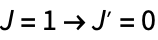atomic transition.

 Define the atomic system.

We want to define an optical field with a modulated frequency, i.e., where the frequency itself is a function of time. We need to be careful when doing this the time dependence of a field with constant frequency ω is written as something like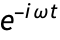, and it is tempting to simply replacein this expression by our chosen time-dependent frequency. However, the factorin the expression for a static field represents the accumulated phase since time zero, i.e., the integral of the frequency from time 0 to. (Conversely, the instantaneous frequency is the derivative of the time-dependent phase.) Thus we should replace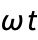by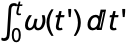.

 Define a linearly ()-polarized optical field with frequency ω and electric-field amplitude written in terms of the Rabi frequency (up to a numerical factor) ΩR.
 Define a modulated frequency with central frequency ω, modulation frequency Ωm, and modulation depth Δm, and integrate to find the total phase accumulated starting at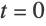.
 Replace ω t with the integrated phase in the definition of the optical field.

In addition to the optical field, we assume that the atoms are subject to a-directed magnetic field with Larmor frequency ΩL.

 Define the Hamiltonian.
 Draw the level diagram, showing optical couplings and Zeeman shifts.

To perform the rotating-wave approximation, we want to transform into a frame that is rotating so as to acquire the same accumulated phase as found above. Since RotatingWaveApproximation takes a frequency as its third argument, we call it with an effective frequency obtained by dividing the total accumulated phase by t. This quantity will then be multiplied by t when the transform matrix is constructed.

 Call RotatingWaveApproximation, which automatically generates an appropriate unitary transform using the supplied effective frequency.

Note that in performing the transformation of the Hamiltonian to the rotating frame, the time derivative of the transform matrix is taken, which introduces a term equal to the derivative of totalPhase into the diagonal term of the excited state energy. This term is precisely the time-dependent frequency that we originally chose. In fact, the effective Hamiltonian that we have obtained is identical to what we would have gotten if we had naively performed the RWA on a Hamiltonian for a static field, and then substituted our chosen expression for instantaneous frequency in place of ω.

In order to generate the density-matrix evolution equations, we also need to describe the relaxation processes in the system. The excited-state spontaneous decay rate is Γ; we also assume a transit relaxation rate of γt.

 Find the matrix describing spontaneous decay and transit relaxation.
 Find the matrix describing repopulation of the ground state due to atom transit and spontaneous decay from the excited state.
 Generate the density-matrix evolution equations.

The DM-evolution equations have explicit time dependence due to the frequency-modulation term. They can be solved numerically using NDSolve if we supply initial conditions and numerical values for all of the parameters.

 Generate initial conditions corresponding to an unpolarized ground state.
 Choose numerical values for all parameters.
 Solve the system of equations with NDSolve and plot the imaginary part of one density-matrix element, corresponding to an optical coherence, as a function of time.

The solution displays an initial transient response, and then settles down to a periodic condition, with period equal to the modulation period 2π/Ωm. If we are primarily interested in this periodic state, it can be much more numerically efficient to solve for it directly, by finding the coefficients of a Fourier expansion of the density matrix, using a Floquet technique.

For the case considered here, the density-matrix-evolution equations can be written in matrix form as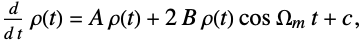whereandare time-independent matrices,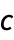is a time-independent vector, and here we consider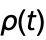to be a real column vector consisting of the real and imaginary parts of the density-matrix elements.

Because the desired solution for the density matrix is periodic, we can perform a Floquet analysis of the system by expandingin harmonics of the modulation frequency: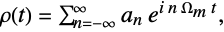where the vectorsare the Fourier coefficients of the expansion, assumed to be time-independent for a periodic solution. (Becauseis real, we have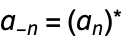.) After substituting this expansion in the evolution equation, the result can be written asDue to the orthogonality of the Fourier basis functions, this equation implies the recursion relationwhere we have defined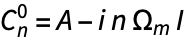, whereis the identity matrix.

The recursion relation can be solved for the coefficientsin various ways. One method, implemented here, finds the solution in terms of a continued fraction involving the matrices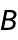and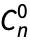. Choosing a cutoff harmonic, above which all Fourier coefficients are assumed to be zero, allows a numerical solution to be obtained.

 EquationsToMatrixRecursion[eqs,vars,Ω,t,n] convert a system of differential equations with explicit sinusoidal time dependence at harmonics of Ω to matrices specifying a recursion relation for the Fourier components with harmonics labeled by n MatrixRSolve[mats,{n,ncut,nreturn},{vars,t}] solve the recursion relation returned by EquationsToMatrixRecursion, assuming Fourier harmonics of order ncut or higher are zero, returning values for harmonics up to nreturn FourierExpandVariables[vars,Ω,t,nmax] write a list of variables in terms of Fourier coefficients up to harmonic nmax FourierExpandAndIntegrate[expr,vars,Ω,t] find the time-average of an expression over an oscillation period, and expresses the result in terms of Fourier coefficients

Solving equations using Floquet analysis and the matrix-continued-fraction method.

 Convert the evolution equations to a matrix recursion expression for the Fourier coefficients.
 Display the form of the generated matrices.
 Solve the recursion relation, using a cutoff harmonic of 10, and return values for the Fourier coefficients up to the ninth harmonic.
 The same DM element plotted above, written in terms of Fourier coefficients up to the ninth harmonic.
 Compare the solutions obtained from NDSolve and MatrixRSolve. After the transients decay, the agreement is quite good.
 Here we compare the solutions using a variable number of Fourier components.

In many experimental situations, lock-in detection is employed, which picks out a single harmonic from the periodic evolution. In this case, we may only need the lowest or the first few harmonics from the solution, even if higher harmonics are required to completely describe the time dependence of the system.

As an example, consider the optical-rotation signal measured with the modulated light beam. First we find the expression for the time-dependent optical rotation in terms of the density-matrix elements. We do this by calling Observables with the effective frequency totalPhase/t. We can set ωω0 in the resulting expression, and set Δm to zero, since it is very small compared to ω. The wavelength λ here contributes here only an overall multiplicative factor, and so can be set to one.